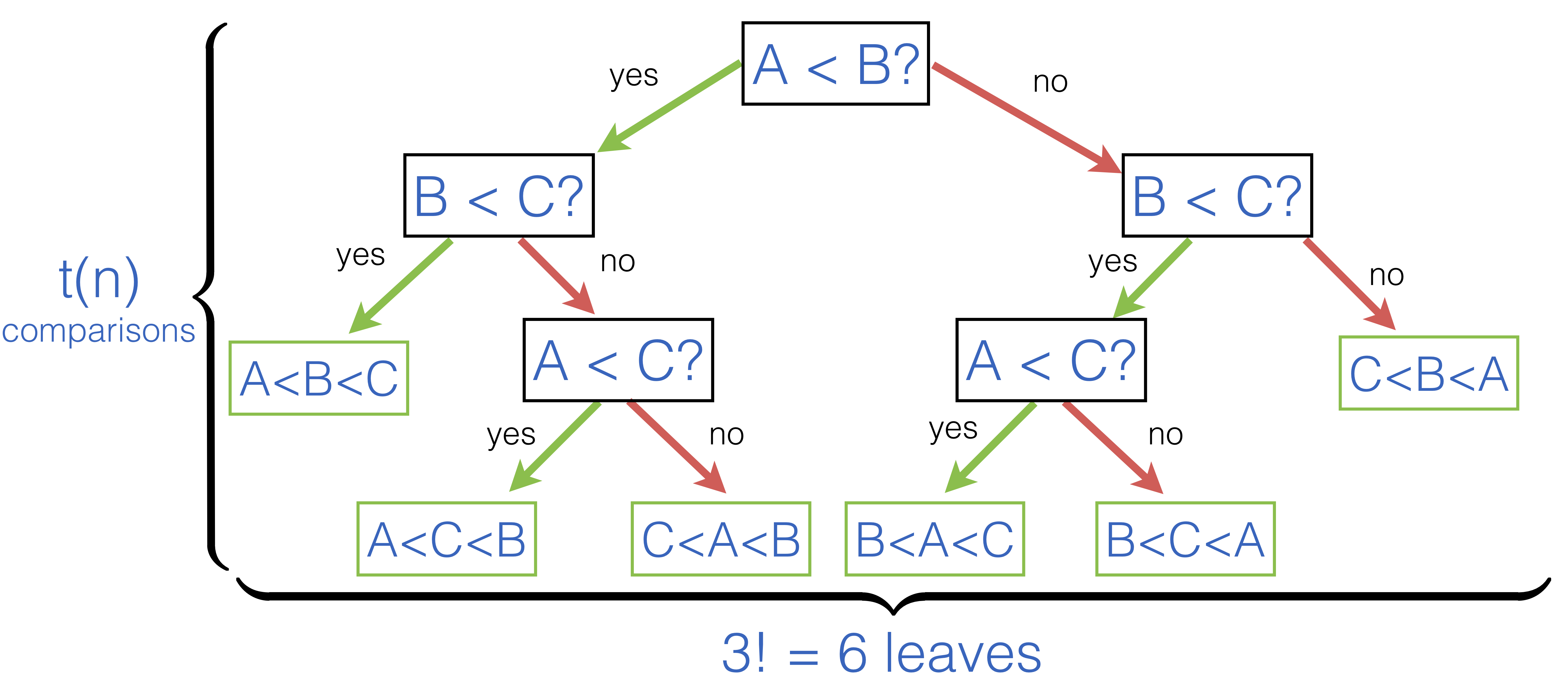# 5.2. Best-Worst Case for Comparison-Based Sorting¶

A strength and a weakness of Comparison-Based Sorting is the fact that the only operation it relies in its implementation is a primitive to compare two elements. This makes it very generic and applicable to diverse classes of data, but also puts a theoretical limit on how fast (in the asymptotic big-O sence) we can sort arrays if we use only comparison.

Let us se what is this theoretical limit. Quicksort, Insertion sort, Merge sort are all comparison-based sorting algorithms: they compare elements pairwise. An “ideal” algorithm will always perform no more than $$t(n)$$ comparisons, for the worst possible case on an array of size $$n$$. What is then $$t(n)$$?

Let us think in the following way. What a sorting delivers is a permutation of an initial array, which is also sorted. A number of possible permutations of $$n$$ elements is $$n!$$, and such an algorithm should find “the right one” by following a path in a binary decision tree, where each node corresponds to comparing just two elements.

As an example, consider the following decision tree for an array of just three elements [|A; B; C|]:Intuitively, by making $$t(n)$$ steps in a decision tree, in its search for the “correctly ordered” permutation, the algorithm should be able to say, which ordering it is. Since the number of reachable leaves in $$t(n)$$ steps is $$2^{t(n)}$$, and the number of possible orderings is $$n!$$, it should be the case that

$2^{t(n)} \geq n!$

To obtain the boundary on $$t(n)$$ we need to solve this inequality. We can do so by first taking tha logarithm of the both sides:

$t(n) \geq \log_2(n!)$

We can then use Stirling’s formula for large $$n$$, which states that $$n! \approx \sqrt{2\pi n}\left(\frac{n}{e}\right)^n$$. Therefore, we obtain

$t(n) \approx n \log_e n = (\log_e 2) n \log_2 n \in O(n \log n)$

With this we establish that the best possible algorithm for sorting arrays using only comparison will be deemed to perform $$O(n \log n)$$ comparisons in the worst case.

The complexity class $$O(n \log n)$$ is so paramount in the study of algorithms that it deserved its own name: computations having this complexity are often referred to as having linearithmic complexity.